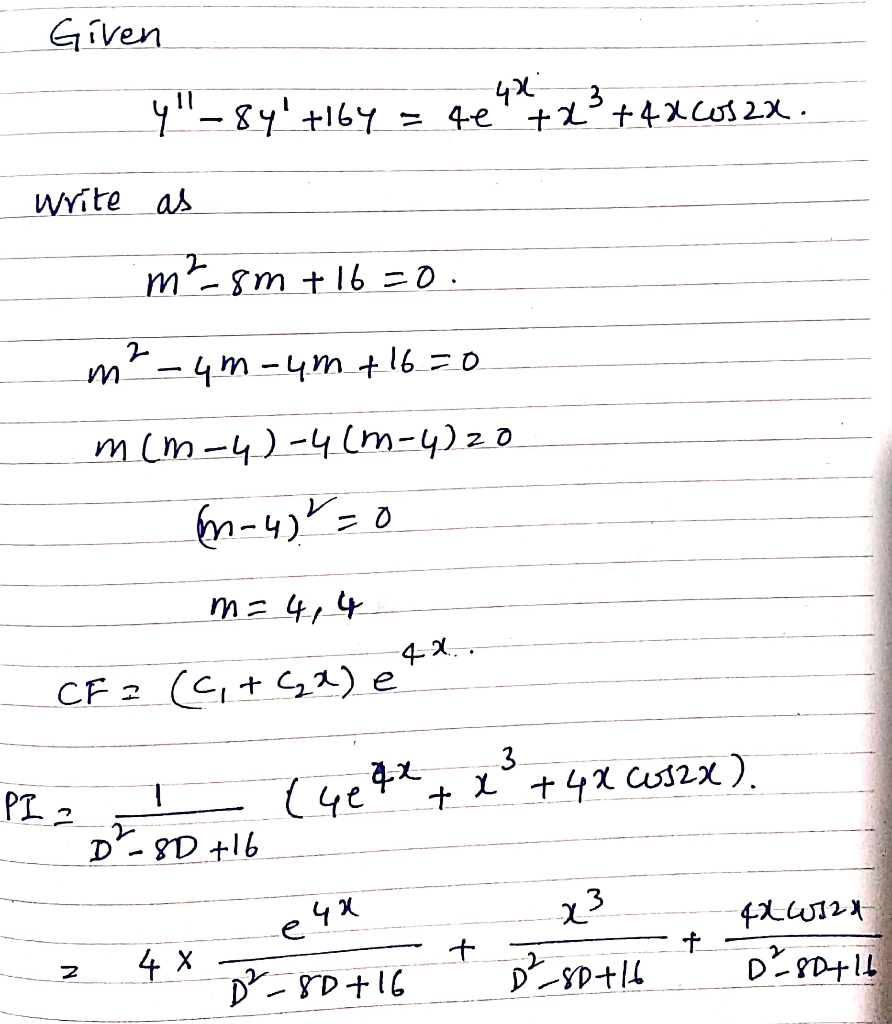# A petroleum refiner company conducts a experiment and produce two products specifically, there are petroleum naphtha and asphalt liquid. By selling the petroleum naphtha to consultant company the petr...

A petroleum refiner company conducts a experiment and produce two products specifically, there are petroleum naphtha and asphalt liquid. By selling the petroleum naphtha to consultant company the petroleum company can gain profits of 4 rupees per litre and, for asphalt liquid per litre the profit is of 3 rupees per litre. Processing each litre of petroleum naphtha requires to spend 3 hours in reactor A of and another 4 hours in reactor B. Also, the processing of one litre of asphalt liquid required to spend 4 and 2 hours, respectively. The time available daily to each of these process of spending in reactor A and reactor B is 12 and 8 hours respectively. Furthermore, it is estimated to produce hardly 3 litres per daily of petroleum product. Determine the number of litres of petroleum naphtha and asphalt liquid to be produced daily in order to maximize gains. (Qa)Formulate the problem accommodating objective and constraint equation? (Qb)Find the desirable values of petroleum naphtha and asphalt liquid variables that satisfy the constraint equation. Code this solution in MATLAB without using linprog command. Should use polyval and polyfit commands. Try to create atleast one user define matlab function Hint: Ignore the inequality in the contraint equations, constructe the equation of curve, plot that line equation within approiate interval values. Use polyval and polyfit commands to fit the plot. Note down the intersect points of lines and substutite values of the intersecting points in the objective equation to obtain the optimal solution (Qc)Find the optimal solution and the values of the contraint variables using linprog command and use this solution to validate the solution of Qb##### Add Answer of: A petroleum refiner company conducts a experiment and produce two products specifically, there are petroleum naphtha and asphalt liquid. By selling the petroleum naphtha to consultant company the petr...
More Homework Help Questions Additional questions in this topic.